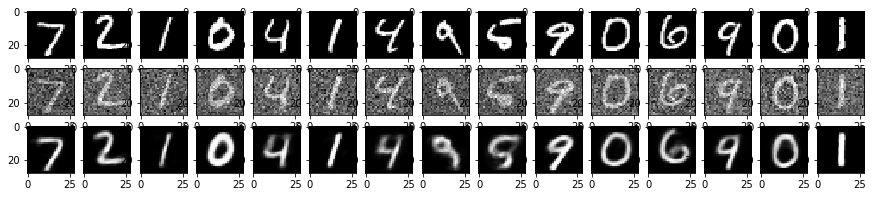# 實作Tensorflow (4)：Autoencoder

Posted on November 18, 2017 in AI.ML. View: 13,287

Autoencoder是一個Neurel Network重要的工具，我個人認為它還漂亮的呈現Neurel Network的強大。

### Autoencoder觀念解析

Autoencoder概念很簡單，就是做資訊的壓縮，概念是這樣的，當我在一層當中使用神經元愈多，可以儲存的資訊量也就愈多，相反的神經元越少，可以儲存的資訊量越少，如果我要使用Neurel Network作資料壓縮的話，我希望的是可以使用比原本更少的資訊量來儲存，如果原本是一張MNIST的圖，有28x28=784個Pixels，所以可以想知，如果我要作壓縮就要使得壓縮後的神經元可以比784個更少。

Encoder的效果等同於做Dimension Reduction，Encoder轉換原本數據到一個新的空間，這個空間可以比原本Features描述的空間更能精簡的描述這群數據，而中間這層Layer的數值Embedding Code就是新空間裡頭的座標，有些時候我們會用這個新空間來判斷每筆Data之間彼此的接近程度。### Autoencoder程式碼

Weight-Elimination L2 Regularizer有這樣的效果原因是這樣的，L2 Regularizer在抑制W的方法是，如果$$W$$的分量大的話就抑制多一點，如果分量小就抑制少一點（因為$$W^2$$微分為一次），所以最後會留下很多不為0的微小分量，不夠Sparse，這樣的Regularization顯然不夠好，L1 Regularizer可以解決這個問題（因為在大部分位置微分為常數），但不幸的是它無法微分，沒辦法作Backpropagation，所以就有了L2 Regularizer的衍生版本，

Weight-elimination L2 regularizer:

$$\sum_{jk} (W_{jk} (ℓ))^2 / [1+ (W_{jk} (ℓ) )^2]$$

  1 2 3 4 5 6 7 8 9 10 11 import random import time import numpy as np import tensorflow as tf import matplotlib.pyplot as plt tf.logging.set_verbosity(tf.logging.ERROR) # Config the matplotlib backend as plotting inline in IPython %matplotlib inline 
  1 2 3 4 5 6 7 8 9 10 11 12 13 14 15 16 17 18 19 20 21 22 23 24 25 26 27 28 29 30 31 32 33 34 35 36 37 38 39 40 41 42 43 44 45 46 47 48 49 50 51 52 53 54 55 56 57 58 59 60 61 62 63 64 65 66 67 68 69 70 71 72 73 74 75 76 77 78 79 80 81 82 83 84 85 86 87 88 89 90 91 92 93 94 95 96 97 98 99 100 101 102 103 104 105 106 107 108 109 110 111 112 113 114 115 116 117 118 119 120 121 122 123 124 125 126 127 128 129 130 131 132 133 134 135 136 137 138 139 140 141 142 143 144 145 146 147 148 149 150 151 152 153 154 155 156 157 158 159 160 161 162 163 164 165 166 167 168 169 170 171 172 173 174 175 176 177 178 179 180 181 182 183 184 185 class Autoencoder: def __init__(self, n_features, learning_rate=0.5, n_hidden=[1000, 500, 250, 2], alpha=0.0): self.n_features = n_features self.weights = None self.biases = None self.graph = tf.Graph() # initialize new grap self.build(n_features, learning_rate, n_hidden, alpha) # building graph self.sess = tf.Session(graph=self.graph) # create session by the graph def build(self, n_features, learning_rate, n_hidden, alpha): with self.graph.as_default(): ### Input self.train_features = tf.placeholder(tf.float32, shape=(None, n_features)) self.train_targets = tf.placeholder(tf.float32, shape=(None, n_features)) ### Optimalization # build neurel network structure and get their predictions and loss self.y_, self.original_loss, _ = self.structure( features=self.train_features, targets=self.train_targets, n_hidden=n_hidden) # regularization loss # weight elimination L2 regularizer self.regularizer = \ tf.reduce_sum([tf.reduce_sum( tf.pow(w, 2)/(1+tf.pow(w, 2))) for w in self.weights.values()]) \ / tf.reduce_sum( [tf.size(w, out_type=tf.float32) for w in self.weights.values()]) # total loss self.loss = self.original_loss + alpha * self.regularizer # define training operation self.optimizer = tf.train.AdamOptimizer(learning_rate=learning_rate) self.train_op = self.optimizer.minimize(self.loss) ### Prediction self.new_features = tf.placeholder(tf.float32, shape=(None, n_features)) self.new_targets = tf.placeholder(tf.float32, shape=(None, n_features)) self.new_y_, self.new_original_loss, self.new_encoder = self.structure( features=self.new_features, targets=self.new_targets, n_hidden=n_hidden) self.new_loss = self.new_original_loss + alpha * self.regularizer ### Initialization self.init_op = tf.global_variables_initializer() def structure(self, features, targets, n_hidden): ### Variable if (not self.weights) and (not self.biases): self.weights = {} self.biases = {} n_encoder = [self.n_features]+n_hidden for i, n in enumerate(n_encoder[:-1]): self.weights['encode{}'.format(i+1)] = \ tf.Variable(tf.truncated_normal( shape=(n, n_encoder[i+1]), stddev=0.1), dtype=tf.float32) self.biases['encode{}'.format(i+1)] = \ tf.Variable(tf.zeros(shape=(n_encoder[i+1])), dtype=tf.float32) n_decoder = list(reversed(n_hidden))+[self.n_features] for i, n in enumerate(n_decoder[:-1]): self.weights['decode{}'.format(i+1)] = \ tf.Variable(tf.truncated_normal( shape=(n, n_decoder[i+1]), stddev=0.1), dtype=tf.float32) self.biases['decode{}'.format(i+1)] = \ tf.Variable(tf.zeros(shape=(n_decoder[i+1])), dtype=tf.float32) ### Structure activation = tf.nn.relu encoder = self.get_dense_layer(features, self.weights['encode1'], self.biases['encode1'], activation=activation) for i in range(1, len(n_hidden)-1): encoder = self.get_dense_layer( encoder, self.weights['encode{}'.format(i+1)], self.biases['encode{}'.format(i+1)], activation=activation, ) encoder = self.get_dense_layer( encoder, self.weights['encode{}'.format(len(n_hidden))], self.biases['encode{}'.format(len(n_hidden))], ) decoder = self.get_dense_layer(encoder, self.weights['decode1'], self.biases['decode1'], activation=activation) for i in range(1, len(n_hidden)-1): decoder = self.get_dense_layer( decoder, self.weights['decode{}'.format(i+1)], self.biases['decode{}'.format(i+1)], activation=activation, ) y_ = self.get_dense_layer( decoder, self.weights['decode{}'.format(len(n_hidden))], self.biases['decode{}'.format(len(n_hidden))], activation=tf.nn.sigmoid, ) loss = tf.reduce_mean(tf.pow(targets - y_, 2)) return (y_, loss, encoder) def get_dense_layer(self, input_layer, weight, bias, activation=None): x = tf.add(tf.matmul(input_layer, weight), bias) if activation: x = activation(x) return x def fit(self, X, Y, epochs=10, validation_data=None, test_data=None, batch_size=None): X = self._check_array(X) Y = self._check_array(Y) N = X.shape random.seed(9000) if not batch_size: batch_size = N self.sess.run(self.init_op) for epoch in range(epochs): print('Epoch %2d/%2d: ' % (epoch+1, epochs)) start_time = time.time() # mini-batch gradient descent index = [i for i in range(N)] random.shuffle(index) while len(index) > 0: index_size = len(index) batch_index = [index.pop() for _ in range(min(batch_size, index_size))] feed_dict = {self.train_features: X[batch_index, :], self.train_targets: Y[batch_index, :]} _, loss = self.sess.run([self.train_op, self.loss], feed_dict=feed_dict) print('[%d/%d] loss = %9.4f ' % (N-len(index), N, loss), end='\r') # evaluate at the end of this epoch msg_valid = '' if validation_data is not None: val_loss = self.evaluate(validation_data, validation_data) msg_valid = ', val_loss = %9.4f' % (val_loss) train_loss = self.evaluate(X, Y) print('[%d/%d] %ds loss = %9.4f %s' % (N, N, time.time()-start_time, train_loss, msg_valid)) if test_data is not None: test_loss = self.evaluate(test_data, test_data) print('test_loss = %9.4f' % (test_loss)) def encode(self, X): X = self._check_array(X) return self.sess.run(self.new_encoder, feed_dict={self.new_features: X}) def predict(self, X): X = self._check_array(X) return self.sess.run(self.new_y_, feed_dict={self.new_features: X}) def evaluate(self, X, Y): X = self._check_array(X) return self.sess.run(self.new_loss, feed_dict={self.new_features: X, self.new_targets: Y}) def _check_array(self, ndarray): ndarray = np.array(ndarray) if len(ndarray.shape) == 1: ndarray = np.reshape(ndarray, (1, ndarray.shape)) return ndarray 

### 測試Autoencoder

 1 2 3 4 5 6 from tensorflow.examples.tutorials.mnist import input_data mnist = input_data.read_data_sets('MNIST_data/', one_hot=True) train_data = mnist.train valid_data = mnist.validation test_data = mnist.test 
 1 2 3 4 5 6 7 8 Successfully downloaded train-images-idx3-ubyte.gz 9912422 bytes. Extracting MNIST_data/train-images-idx3-ubyte.gz Successfully downloaded train-labels-idx1-ubyte.gz 28881 bytes. Extracting MNIST_data/train-labels-idx1-ubyte.gz Successfully downloaded t10k-images-idx3-ubyte.gz 1648877 bytes. Extracting MNIST_data/t10k-images-idx3-ubyte.gz Successfully downloaded t10k-labels-idx1-ubyte.gz 4542 bytes. Extracting MNIST_data/t10k-labels-idx1-ubyte.gz 
  1 2 3 4 5 6 7 8 9 10 11 12 13 14 15 16 17 18 19 20 21 22 model_1 = Autoencoder( n_features=28*28, learning_rate= 0.0005, n_hidden=[512, 32, 4], alpha=0.0, ) model_1.fit( X=train_data.images, Y=train_data.images, epochs=20, validation_data=(valid_data.images, valid_data.images), test_data=(test_data.images, test_data.images), batch_size = 8, ) fig, axis = plt.subplots(2, 15, figsize=(15, 2)) for i in range(0, 15): img_original = np.reshape(test_data.images[i], (28, 28)) axis[i].imshow(img_original, cmap='gray') img = np.reshape(model_1.predict(test_data.images[i]), (28, 28)) axis[i].imshow(img, cmap='gray') plt.show() 
  1 2 3 4 5 6 7 8 9 10 11 12 13 14 15 16 17 18 19 20 21 22 23 24 25 26 27 28 29 30 31 32 33 34 35 36 37 38 39 40 41 Epoch 1/20: [55000/55000] 15s loss = 0.0332 , val_loss = 0.0327 Epoch 2/20: [55000/55000] 14s loss = 0.0303 , val_loss = 0.0299 Epoch 3/20: [55000/55000] 14s loss = 0.0288 , val_loss = 0.0285 Epoch 4/20: [55000/55000] 14s loss = 0.0278 , val_loss = 0.0275 Epoch 5/20: [55000/55000] 14s loss = 0.0272 , val_loss = 0.0271 Epoch 6/20: [55000/55000] 14s loss = 0.0267 , val_loss = 0.0268 Epoch 7/20: [55000/55000] 14s loss = 0.0263 , val_loss = 0.0264 Epoch 8/20: [55000/55000] 14s loss = 0.0259 , val_loss = 0.0262 Epoch 9/20: [55000/55000] 14s loss = 0.0256 , val_loss = 0.0259 Epoch 10/20: [55000/55000] 14s loss = 0.0254 , val_loss = 0.0257 Epoch 11/20: [55000/55000] 14s loss = 0.0252 , val_loss = 0.0257 Epoch 12/20: [55000/55000] 14s loss = 0.0250 , val_loss = 0.0256 Epoch 13/20: [55000/55000] 14s loss = 0.0248 , val_loss = 0.0255 Epoch 14/20: [55000/55000] 14s loss = 0.0247 , val_loss = 0.0254 Epoch 15/20: [55000/55000] 14s loss = 0.0245 , val_loss = 0.0253 Epoch 16/20: [55000/55000] 14s loss = 0.0244 , val_loss = 0.0253 Epoch 17/20: [55000/55000] 14s loss = 0.0242 , val_loss = 0.0251 Epoch 18/20: [55000/55000] 14s loss = 0.0243 , val_loss = 0.0252 Epoch 19/20: [55000/55000] 14s loss = 0.0240 , val_loss = 0.0251 Epoch 20/20: [55000/55000] 14s loss = 0.0239 , val_loss = 0.0250 test_loss = 0.0256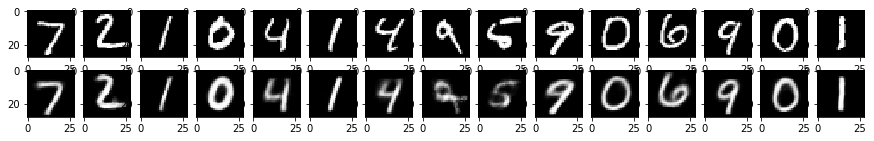1 2 3 4 5 6 7 8 9 10 11 12 13 14 15 16 17 18 19 20 21 22 model_2 = Autoencoder( n_features=28*28, learning_rate= 0.0005, n_hidden=[512, 32, 4], alpha=0.001, ) model_2.fit( X=train_data.images, Y=train_data.images, epochs=20, validation_data=(valid_data.images, valid_data.images), test_data=(test_data.images, test_data.images), batch_size = 8, ) fig, axis = plt.subplots(2, 15, figsize=(15, 2)) for i in range(0, 15): img_original = np.reshape(test_data.images[i], (28, 28)) axis[i].imshow(img_original, cmap='gray') img = np.reshape(model_2.predict(test_data.images[i]), (28, 28)) axis[i].imshow(img, cmap='gray') plt.show() 
  1 2 3 4 5 6 7 8 9 10 11 12 13 14 15 16 17 18 19 20 21 22 23 24 25 26 27 28 29 30 31 32 33 34 35 36 37 38 39 40 41 Epoch 1/20: [55000/55000] 18s loss = 0.0320 , val_loss = 0.0316 Epoch 2/20: [55000/55000] 17s loss = 0.0296 , val_loss = 0.0294 Epoch 3/20: [55000/55000] 17s loss = 0.0285 , val_loss = 0.0283 Epoch 4/20: [55000/55000] 17s loss = 0.0277 , val_loss = 0.0277 Epoch 5/20: [55000/55000] 17s loss = 0.0271 , val_loss = 0.0272 Epoch 6/20: [55000/55000] 17s loss = 0.0266 , val_loss = 0.0268 Epoch 7/20: [55000/55000] 17s loss = 0.0262 , val_loss = 0.0265 Epoch 8/20: [55000/55000] 17s loss = 0.0260 , val_loss = 0.0264 Epoch 9/20: [55000/55000] 17s loss = 0.0257 , val_loss = 0.0261 Epoch 10/20: [55000/55000] 17s loss = 0.0256 , val_loss = 0.0260 Epoch 11/20: [55000/55000] 17s loss = 0.0255 , val_loss = 0.0260 Epoch 12/20: [55000/55000] 17s loss = 0.0251 , val_loss = 0.0257 Epoch 13/20: [55000/55000] 17s loss = 0.0249 , val_loss = 0.0256 Epoch 14/20: [55000/55000] 17s loss = 0.0248 , val_loss = 0.0256 Epoch 15/20: [55000/55000] 18s loss = 0.0247 , val_loss = 0.0255 Epoch 16/20: [55000/55000] 17s loss = 0.0245 , val_loss = 0.0253 Epoch 17/20: [55000/55000] 17s loss = 0.0243 , val_loss = 0.0252 Epoch 18/20: [55000/55000] 17s loss = 0.0242 , val_loss = 0.0252 Epoch 19/20: [55000/55000] 17s loss = 0.0242 , val_loss = 0.0252 Epoch 20/20: [55000/55000] 17s loss = 0.0240 , val_loss = 0.0251 test_loss = 0.0256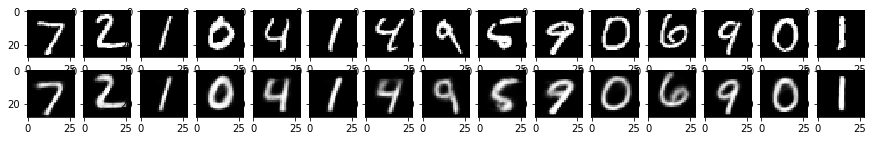### 壓縮碼Code與視覺化

  1 2 3 4 5 6 7 8 9 10 11 12 13 14 # get code encode = model_2.encode(test_data.images) # PCA 2D visualization from sklearn.decomposition import PCA pca = PCA(n_components=2) X = pca.fit_transform(encode) Y = np.argmax(test_data.labels, axis=1) # plot plt.figure(figsize=(10, 8)) plt.scatter(X[:, 0], X[:, 1], c=Y) plt.colorbar() plt.show()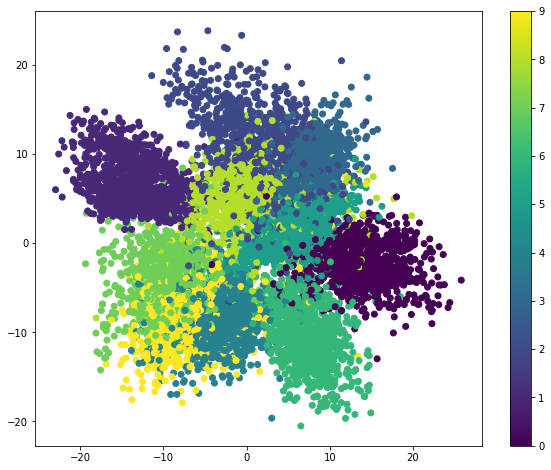1 2 3 4 5 6 7 8 9 10 11 12 13 14 # get code encode = model_2.encode(test_data.images) # TSNE 2D visualization from sklearn.manifold import TSNE tsne = TSNE(n_components=2) X_embedded = tsne.fit_transform(encode) Y = np.argmax(test_data.labels, axis=1) # plot plt.figure(figsize=(10, 8)) plt.scatter(X_embedded[:, 0], X_embedded[:, 1], c=Y) plt.colorbar() plt.show()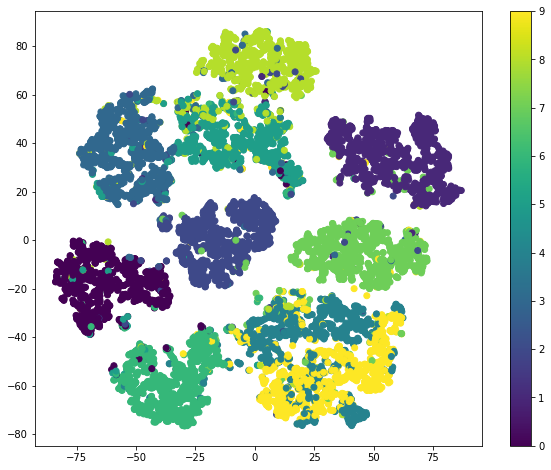### 去雜訊(De-noise) Autoencoder

  1 2 3 4 5 6 7 8 9 10 11 12 13 14 15 def add_noise(ndarr): noise_factor = 0.3 noisy_ndarr = ndarr + noise_factor * np.random.normal(loc=0.0, scale=1.0, size=ndarr.shape) return noisy_ndarr noisy_train_img = add_noise(train_data.images) noisy_valid_img = add_noise(valid_data.images) noisy_test_img = add_noise(test_data.images) fig, axis = plt.subplots(1, 15, figsize=(15, 1)) for i in range(0, 15): img = np.reshape(noisy_train_img[i], (28, 28)) axis[i].imshow(img, cmap='gray') plt.show()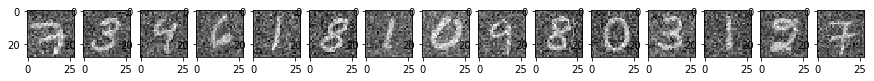1 2 3 4 5 6 7 8 9 10 11 12 13 14 15 16 17 18 19 20 21 22 23 24 denoise_model = Autoencoder( n_features=28*28, learning_rate= 0.0003, n_hidden=[512, 32, 4], alpha=1.0, ) denoise_model.fit( X=noisy_train_img, Y=train_data.images, epochs=20, validation_data=(noisy_valid_img, valid_data.images), test_data=(noisy_test_img, test_data.images), batch_size = 8, ) fig, axis = plt.subplots(3, 15, figsize=(15, 3)) for i in range(0, 15): img_original = np.reshape(test_data.images[i], (28, 28)) axis[i].imshow(img_original, cmap='gray') img_noisy = np.reshape(noisy_test_img[i], (28, 28)) axis[i].imshow(img_noisy, cmap='gray') img = np.reshape(denoise_model.predict(noisy_test_img[i]), (28, 28)) axis[i].imshow(img, cmap='gray') plt.show() 
  1 2 3 4 5 6 7 8 9 10 11 12 13 14 15 16 17 18 19 20 21 22 23 24 25 26 27 28 29 30 31 32 33 34 35 36 37 38 39 40 41 Epoch 1/20: [55000/55000] 18s loss = 0.0393 , val_loss = 0.0389 Epoch 2/20: [55000/55000] 17s loss = 0.0356 , val_loss = 0.0355 Epoch 3/20: [55000/55000] 17s loss = 0.0341 , val_loss = 0.0341 Epoch 4/20: [55000/55000] 17s loss = 0.0327 , val_loss = 0.0329 Epoch 5/20: [55000/55000] 17s loss = 0.0320 , val_loss = 0.0324 Epoch 6/20: [55000/55000] 17s loss = 0.0314 , val_loss = 0.0320 Epoch 7/20: [55000/55000] 17s loss = 0.0309 , val_loss = 0.0316 Epoch 8/20: [55000/55000] 18s loss = 0.0307 , val_loss = 0.0315 Epoch 9/20: [55000/55000] 17s loss = 0.0302 , val_loss = 0.0312 Epoch 10/20: [55000/55000] 17s loss = 0.0299 , val_loss = 0.0309 Epoch 11/20: [55000/55000] 17s loss = 0.0298 , val_loss = 0.0310 Epoch 12/20: [55000/55000] 17s loss = 0.0296 , val_loss = 0.0308 Epoch 13/20: [55000/55000] 17s loss = 0.0293 , val_loss = 0.0307 Epoch 14/20: [55000/55000] 17s loss = 0.0291 , val_loss = 0.0306 Epoch 15/20: [55000/55000] 17s loss = 0.0290 , val_loss = 0.0305 Epoch 16/20: [55000/55000] 17s loss = 0.0287 , val_loss = 0.0304 Epoch 17/20: [55000/55000] 17s loss = 0.0287 , val_loss = 0.0304 Epoch 18/20: [55000/55000] 17s loss = 0.0287 , val_loss = 0.0304 Epoch 19/20: [55000/55000] 17s loss = 0.0286 , val_loss = 0.0304 Epoch 20/20: [55000/55000] 17s loss = 0.0285 , val_loss = 0.0303 test_loss = 0.0309